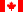Canadian Version Return to Calculator : Help - Contents

### t = -(T1/2/0.693) * ln(N/No)

Use this equation to find out how long it will take for a given initial activity of radioactive material to decay to a given final activity.

To use the equation:
The information required by the equation is the initial activity (No), the final activity (N), and the half-life of the isotope (T½).

1. Select the isotope from the list at the top of the page; this provides the equation with the isotope's half-life. If your isotope is not on the list, you can still use the equation if you manually enter the half-life in the Half-life textbox at the top of the page. You can get half-life values here.
2. Enter the initial activity, No. It is not necessary to worry about units here as long as the units for the final activity are the same.
3. Enter the final activity, N.
4. Click the Calculate button and the decay time is displayed. The time unit will always be the same as the half-life's time unit. If you enter the activities in the wrong order, the answer received will be 0.